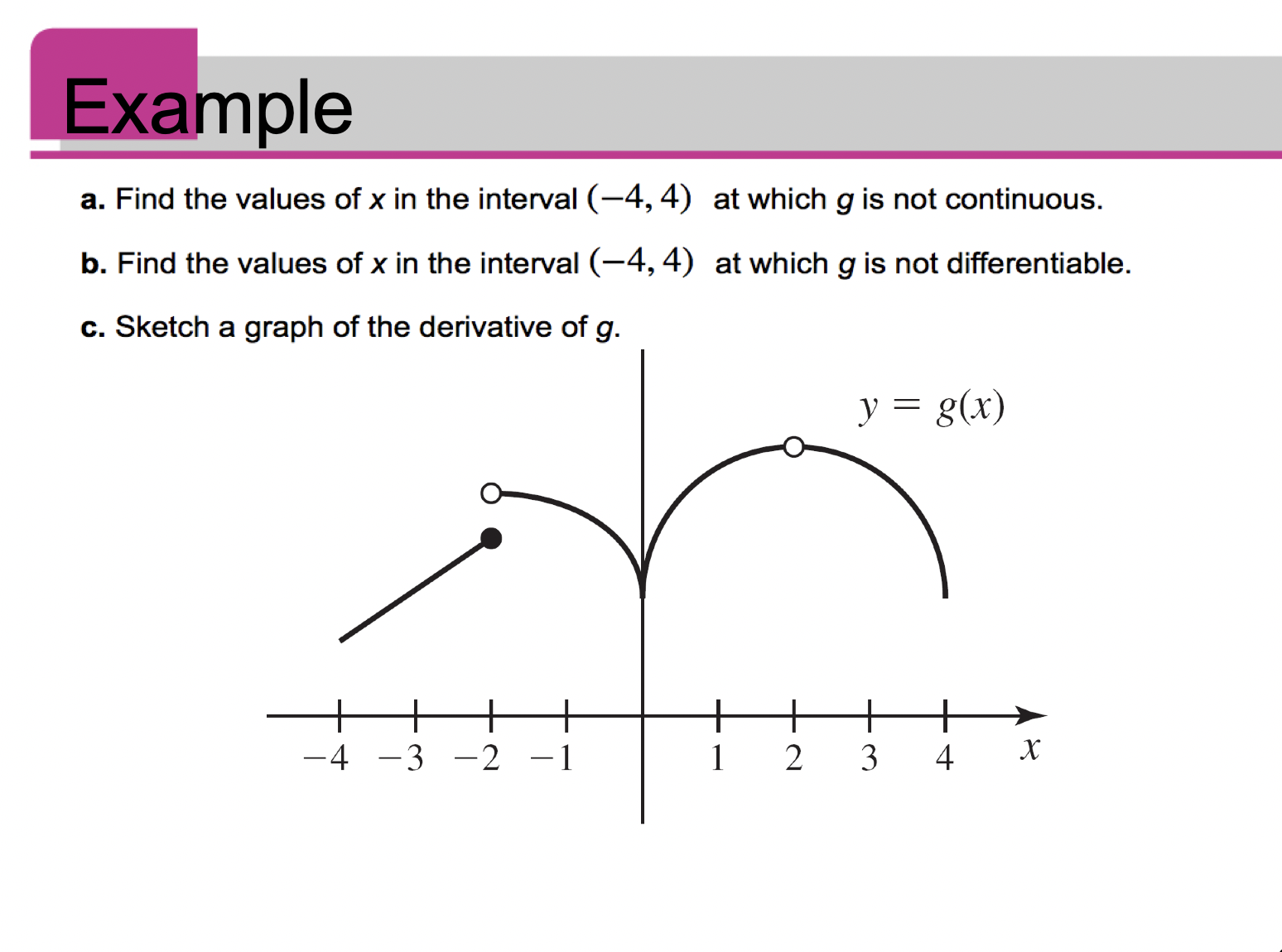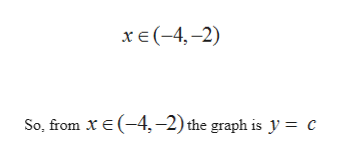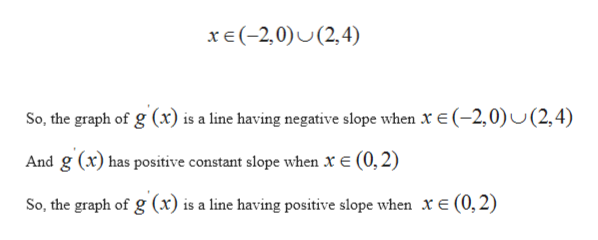# Examplea. Find the values of x in the interval (-4, 4) at which g is not continuousb. Find the values of x in the interval (-4, 4) at which g is not differentiable.c. Sketch a graph of the derivative of g.y g(x)++х-4 -3 -2 -1234

Question

please help with letter c. i am having trouble drawing the graph. i know there is supposed to be a vertical asymptote at y=0  but im not sure how that will impact what the rest of the graph looks likehelp_outlineImage TranscriptioncloseExample a. Find the values of x in the interval (-4, 4) at which g is not continuous b. Find the values of x in the interval (-4, 4) at which g is not differentiable. c. Sketch a graph of the derivative of g. y g(x) + + х -4 -3 -2 -1 2 3 4 fullscreen
check_circle

Step 1

As the derivative of any function is the slope of that function at any point

Step 2

Slope of the g(x) is zero whenhelp_outlineImage Transcriptioncloseх е(-4,-2) So, from x E (-4, -2) the graph is y = c fullscreen
Step 3

And graph  has a negativ...help_outlineImage Transcriptionclosexe(-2,0)(2,4) So, the graph of g (x) is a line having negative slope when x e(-2,0)U(2,4) And g (x) has positive constant slope when x E (0, 2) So, the graph of g (x) is a line having positive slope when xe(0,2) fullscreen

### Want to see the full answer?

See Solution

#### Want to see this answer and more?

Solutions are written by subject experts who are available 24/7. Questions are typically answered within 1 hour.*

See Solution
*Response times may vary by subject and question.
Tagged in

### Calculus